Question

# Find the exact value of the given expression.

Find the exact value of the given expression.

sin(2cos-1 24/25)

_______

Find the exact value of the given expression.

sec(2 sin-1 1/8)

_______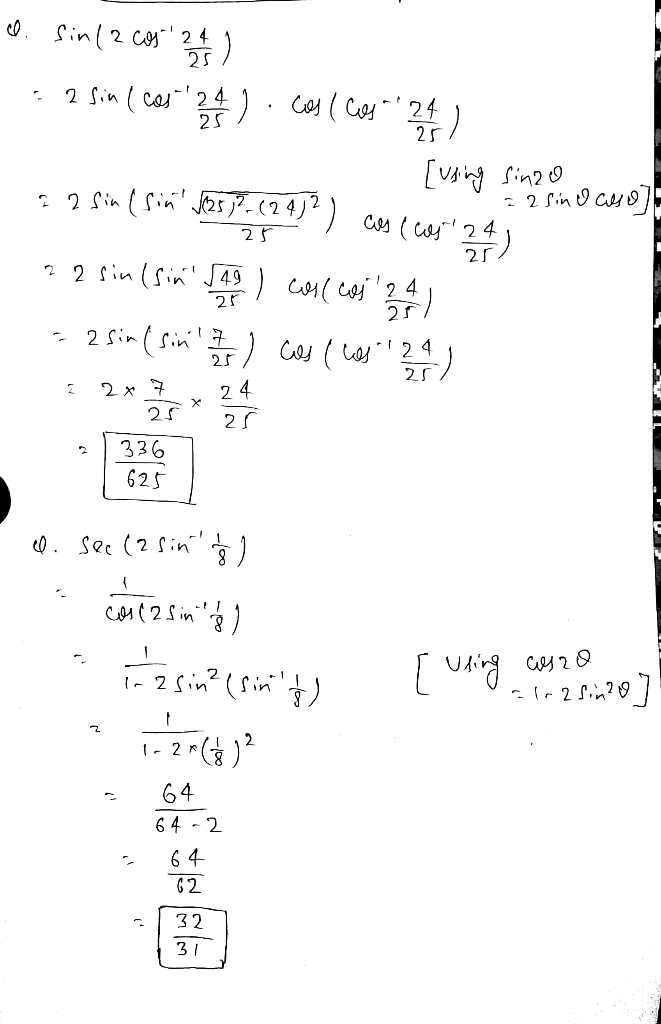9.

sin(2 cos^(-1) 24 / 25)

Let  cos^(-1) 24 / 25 = x

=> cos(x) = 24/25  .

So,

sin(2 cos^(-1) 24 / 25)

= sin(2x)

= 2 sin(x) cos(x)

= 2 sqrt(1 - coś^2 x) coś(x)

= 2 sqrt( 1 - (24/25)^2) * 24 / 25

= 2 * sqrt((625-576) / 625) * 24 / 25

= 2 * sqrt(49 / 625) * 24 / 25

= 2* 7 / 25 * 24 / 25

10.

sec(2 sin^(-1) 1 / 8 )

Let sin((-1) 1 / 8 = x

=> 1/8 = sin(x)

=> cos(x) = sqrt( 1 - (1/8)^2) = sqrt(63 / 64)

=> cos^2 (x) = 63/64

Now,

sec(2 sin^(-1) 1 / 8)

= sec (2x)

= 1/cos(2x)

= 1 / (2cos^2 (x) - 1)

= 1 / (2*63/64 - 1)

= 1 / (62 / 64)

= 64/62

#### Earn Coins

Coins can be redeemed for fabulous gifts.

Similar Homework Help Questions
• ### Find the exact value of the expression.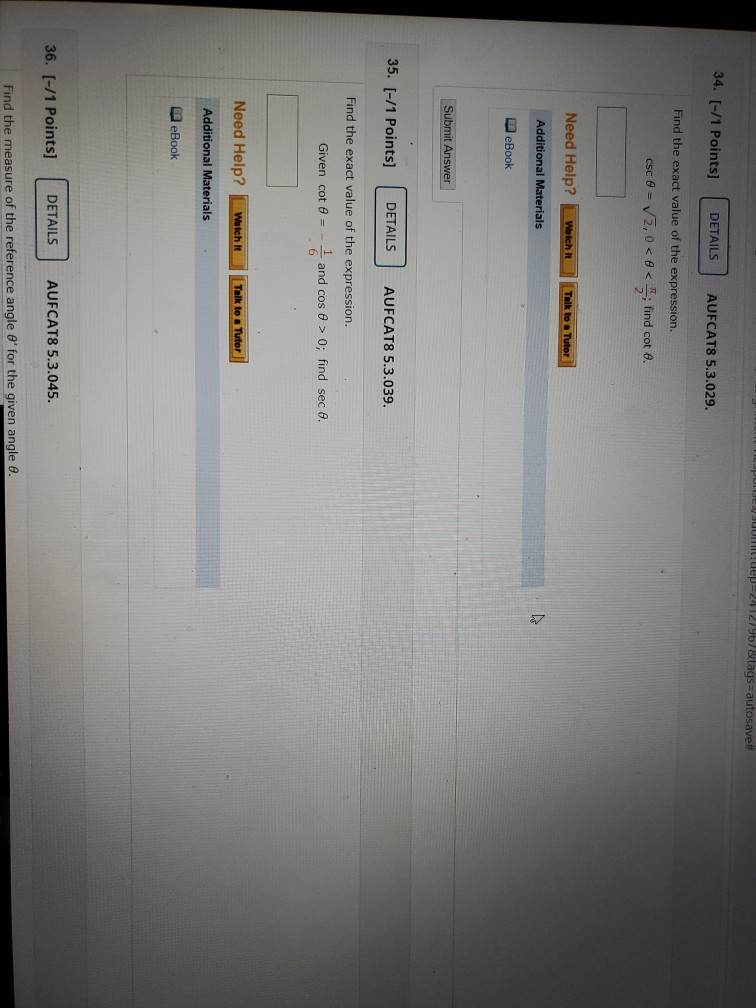Find the exact value of the expression.$$\csc \theta=\sqrt{2}, 0<\theta<\frac{\pi}{2} ; \text { find } \cot \theta$$Find the exact value of the expression.Given $$\cot \theta=-\frac{1}{6}$$ and $$\cos \theta>0$$; find $$\sec \theta$$.

• ### Use the given information to find the exact value of the expression. sin a= 20 29...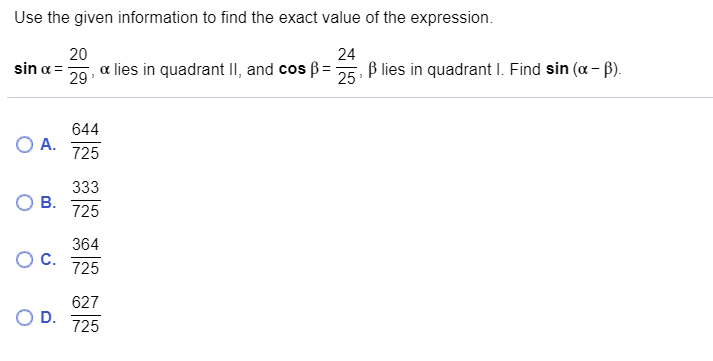Use the given information to find the exact value of the expression. sin a= 20 29 a lies in quadrant II, and cos 24 25 Blies in quadrant I. Find sin (a - ). O A. 644 725 ОВ. 333 725 C. 364 725 627 D. 725

• ### QUESTION 2 Find the exact value under the given conditions. sin a 3- - , <a...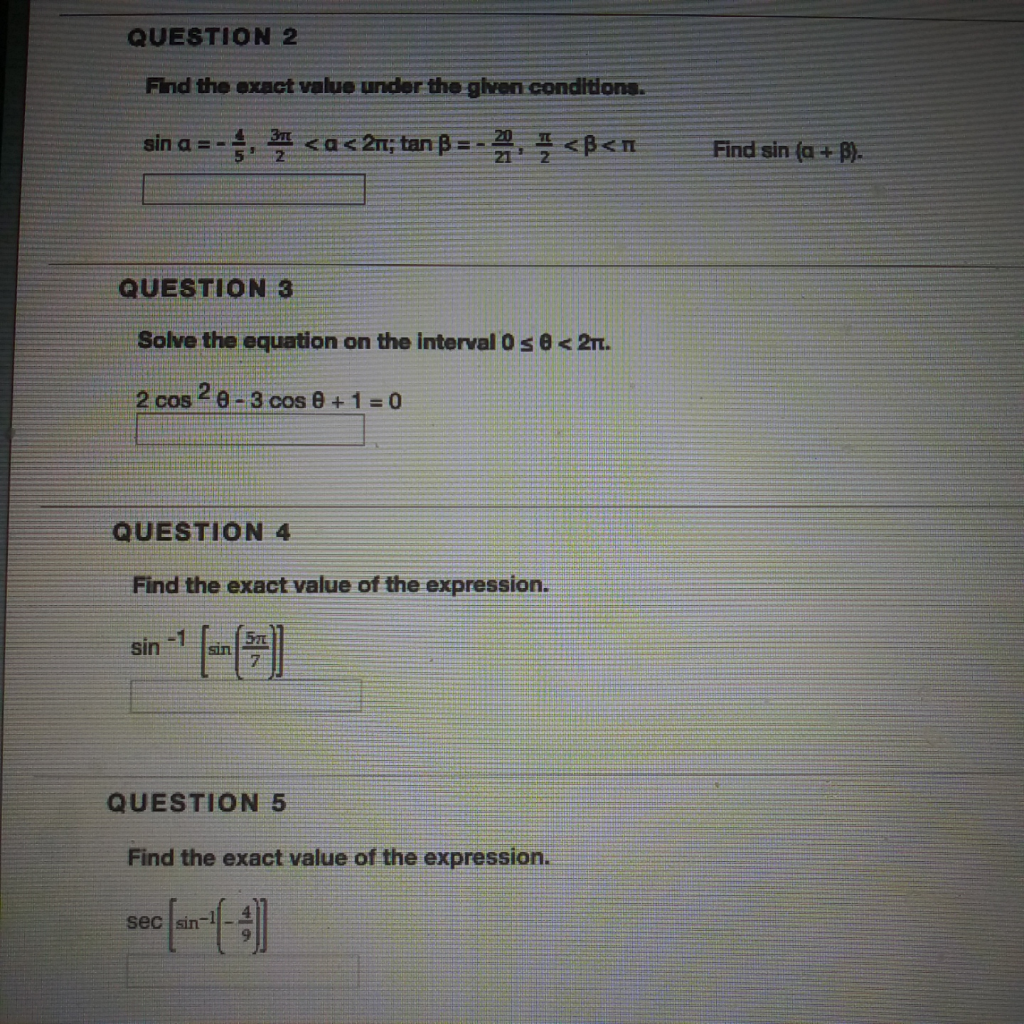QUESTION 2 Find the exact value under the given conditions. sin a 3- - , <a < 211; tan B =-<B<n Find sin (a + B). QUESTION 3 Solve the equation on the interval 0 s < 21. 2 cos 2 0 - 3 cose + 1 = 0 QUESTION 4 Find the exact value of the expression. 577 sin SUN QUESTION 5 Find the exact value of the expression. sec sin

• ### Use the given information to find the exact value of the expression...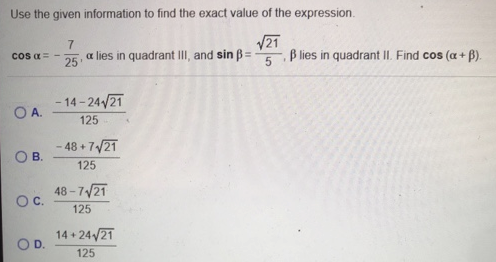Use the given information to find the exact value of the expression$$\cos \alpha=-\frac{7}{25}, \alpha$$ lies in quadrant $$I I I$$, and $$\sin \beta=\frac{\sqrt{21}}{5}, \beta$$ lies in quadrant II . Find $$\cos (\alpha+\beta)$$.A. $$\frac{-14-24 \sqrt{21}}{125}$$B. $$\frac{-48+7 \sqrt{21}}{125}$$c. $$\frac{48-7 \sqrt{21}}{125}$$D. $$\frac{14+24 \sqrt{21}}{125}$$

• ### Problem D Find the exact value of each expression. If the answer is an angle, the...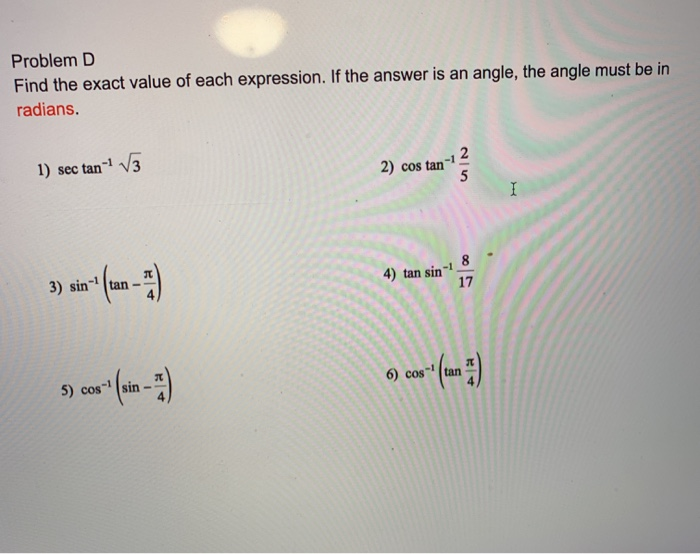Problem D Find the exact value of each expression. If the answer is an angle, the angle must be in radians. 1) sec tan-- V3 2) cos tan 2 5 I 8 4) tan sin 3) sintan 17 6) cos- 5) cos(sin

• ### 16.Find the exact value of the expression in radians and degrees. Find the exact value of...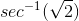16.Find the exact value of the expression in radians and degrees. Find the exact value of the expression in radians = Type an exact answer, using as needed. Use integers or fractions for any numbers in the expression. Type your answer in radians. Find the exact value of the expression in degrees. Type your answer in degrees We were unable to transcribe this imageWe were unable to transcribe this imageWe were unable to transcribe this imagesec 0 -1 (V2)

• ### 1- 2- Question 17 Find the exact value of the expression using the provided information. Find...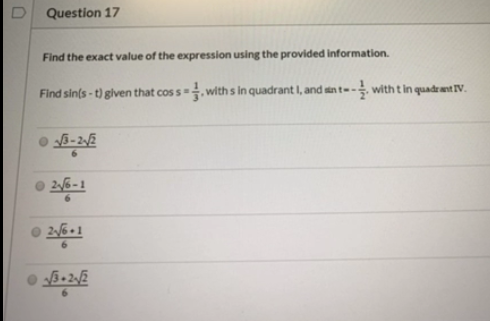1- 2- Question 17 Find the exact value of the expression using the provided information. Find sin(s - t) given that coss - withs in quadrant I, and sunt- -- with t in quadrat IV. 15-212 216-1 2/6.1 15.212 Question 19 Determine the equation of the graph U Oy-2 Scx y--sec 2x y=-2 secx ye-cc2x

• ### w Find the exact value of the trigonometric expression given than sin u = - where...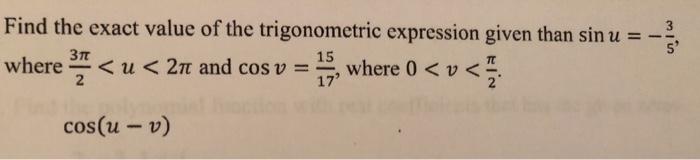w Find the exact value of the trigonometric expression given than sin u = - where 3 < <u<2nt and cos v = - 15, where 0 < v cos(u - v)

• ### 5. (12pts) Find the exact value of the given expression in radians. a. sin-(sin b. cos-(cos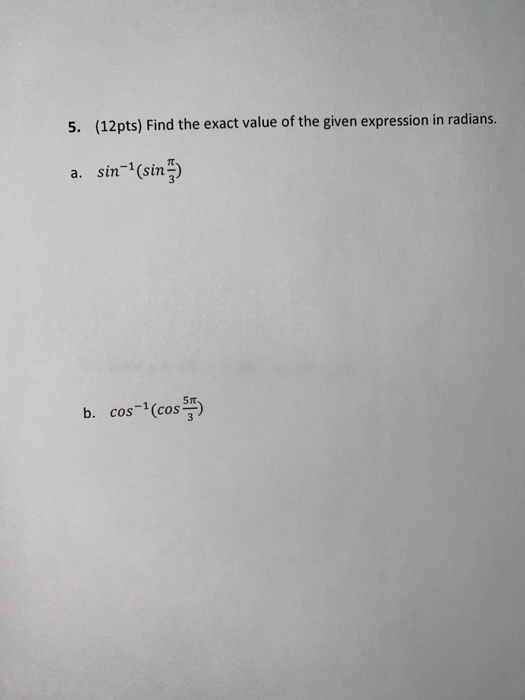5. (12pts) Find the exact value of the given expression in radians. a. sin-(sin b. cos-(cos

• ### 1-17 u ule exact value of the expression 1) cos? 30+ cos? 60 2) cot 45-tan...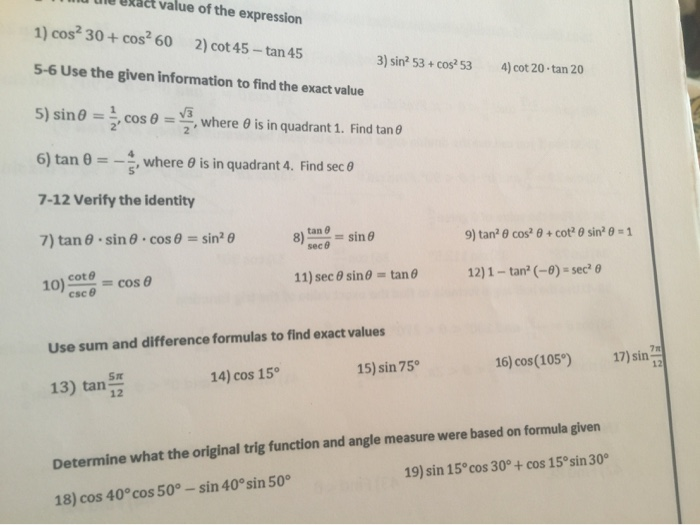1-17 u ule exact value of the expression 1) cos? 30+ cos? 60 2) cot 45-tan 45 5-6 Use the given information to find the exact value 3) sin? 53 + cos2 53 4) cot 20 -tan 20 5) sin = , where is in quadrant 1. Find tan 6) tan 0 = - , where is in quadrant 4. Find sec 7-12 Verify the identity 7) tan sin cos 0 = sin? 8) tane = sine 9) tancos? +...